Jarvis' Blog (总有美丽的风景让人流连) 总有美丽的风景让人流连

# 循环神经网络 (Recurrent Neural Network, RNN)

2018-01-24
2019-11-18
Jarvis
Post

## 1. 循环神经网络 (Recurrent Neural Network, RNN)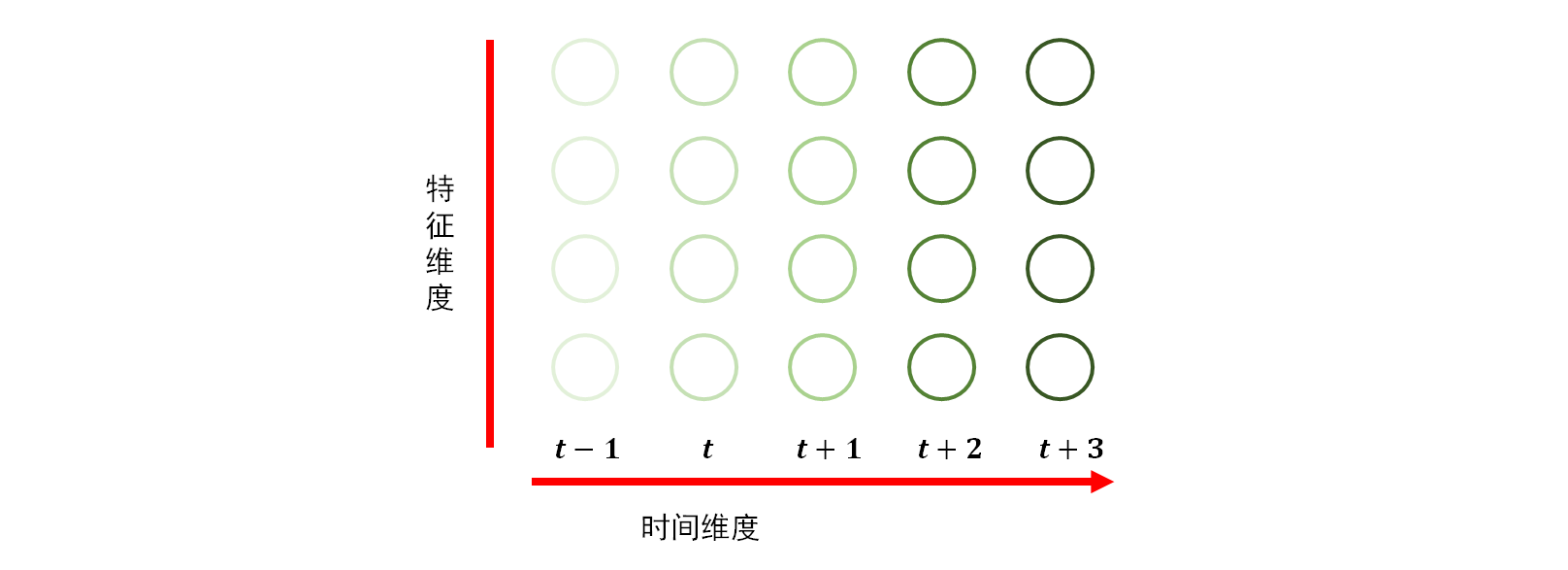$h_t = \sigma(W_{hx}x_t + W_{hh}h_{t-1}).$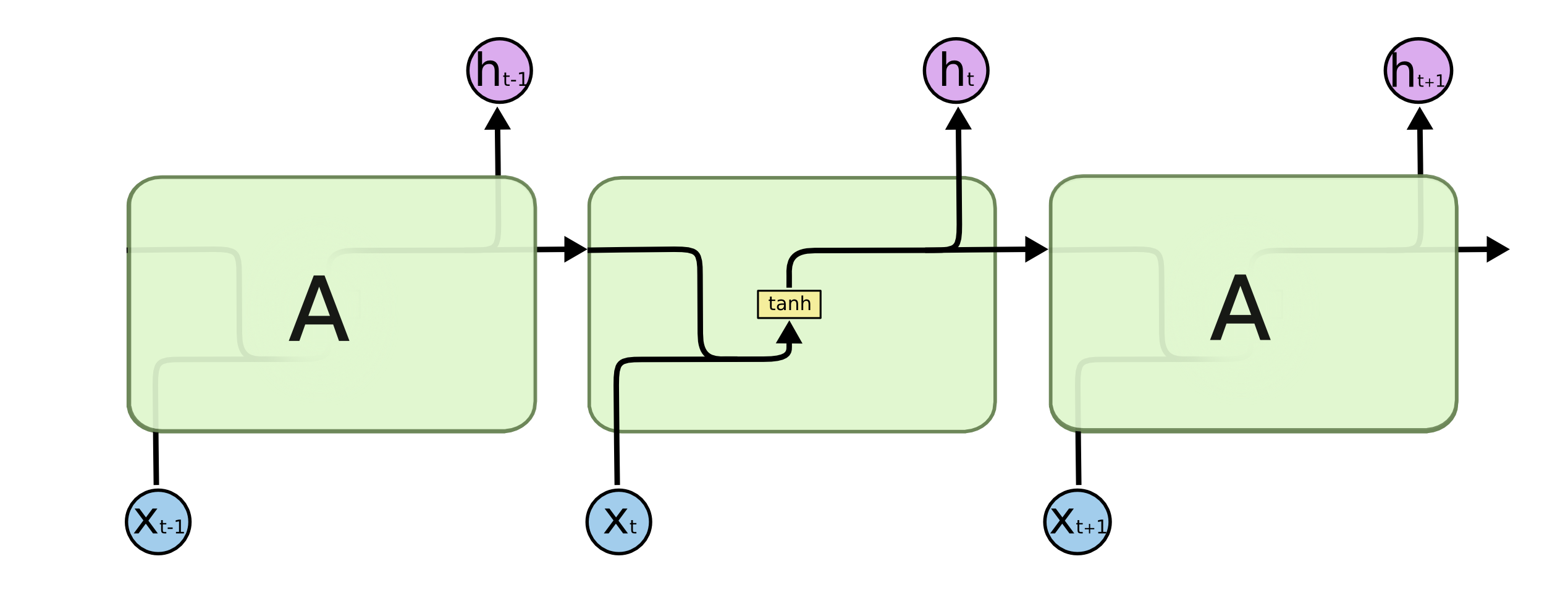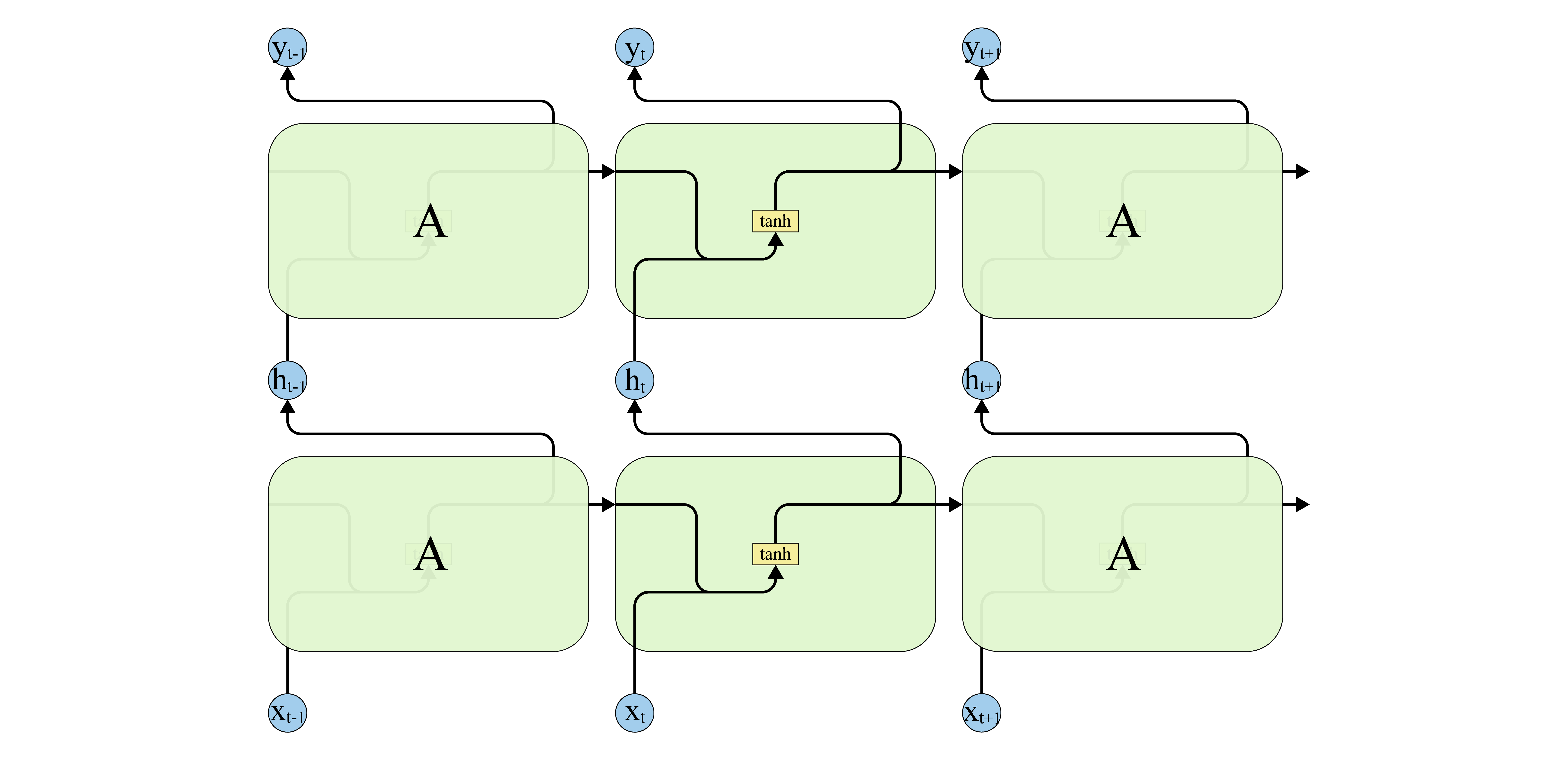RNN 的输入通常表示成嵌入 (embedding)的形式, 即构造一个查询表 (lookup table), 把输入序列的每个时刻的特征向量通过查询表转为一个等长的向量. 从而一个序列的形状变为 [num_time_steps, embedding_size].

### 1.1 RNN 的应用

RNN 可以根据输入序列的长度和输出序列的长度分为三大类.

• 多对一: 常用于情感分析, 文本分类
• 一对多: Image Caption
• 多对多: 机器翻译
• 一对一: 退化为 MLP

### 1.2 RNN 的局限性

RNN 也存在一些缺陷:

• RNN 可以很好的学习序列中邻近时间步数据点(短期)之间的关系, 但对于长期依赖会变得不稳定.
• RNN 可以把固定长度的输入序列映射到指定长度的输出序列, 但不能动态地根据输入决定输出多长的序列.

## 2. 长短期记忆 (Long Short Term Memory, LSTM)1

LSTM 和通常的 CNN 一样为一个循环单元的结构, 但是与 RNN 仅有一个 tanh 激活层不同, LSTM 中包含了更复杂的四层网络的结构设计, 并且四层网络相互耦合, 如下图所示.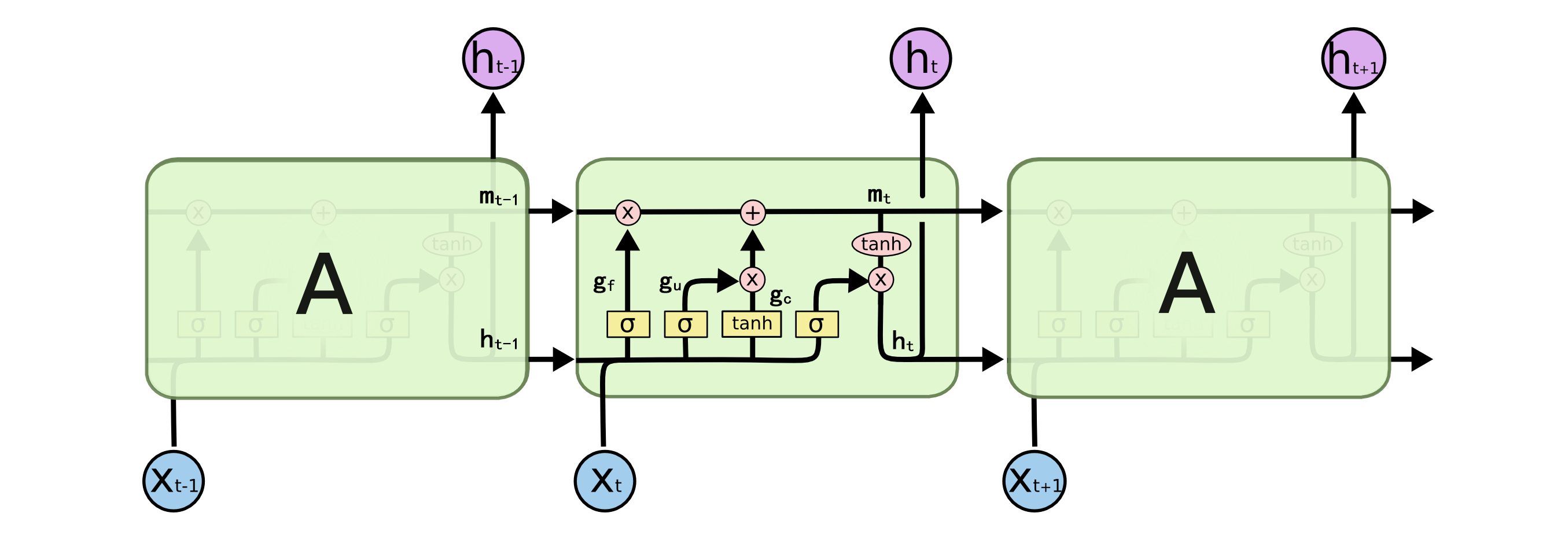### 2.1 LSTM 的核心思想

LSTM 相比于 RNN, 关键在于引入了单元状态(state) $$C$$ —— 横穿下图顶部的直线.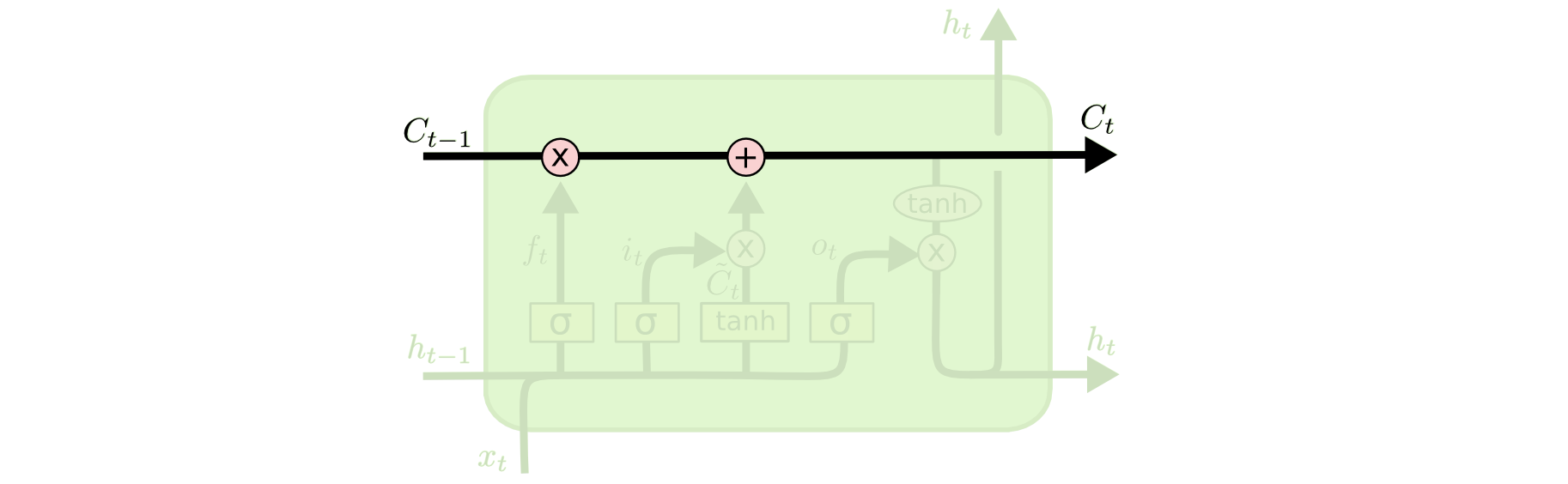LSTM 可以通过门(gate)来控制向单元状态中增加信息或减少信息. 门由一个 $$sigmoid$$ 函数和一个乘法运算符组成, 如下图所示.$$sigmoid$$ 层输出的值在 $$[0, 1]$$ 之间, 控制了信息的通过量. 越接近 0, 则表明不允许信息通过(从而形成遗忘); 越接近 1, 则表明允许信息全部通过(从而形成记忆).

### 2.2 LSTM 单元解析

LSTM 单元在每个时间步需要注意三个向量:

• 输入的特征向量 $$x_t$$
• 上一步输出的特征向量 $$h_{t-1}$$
• 上一步结束后的单元状态 $$C_{t-1}$$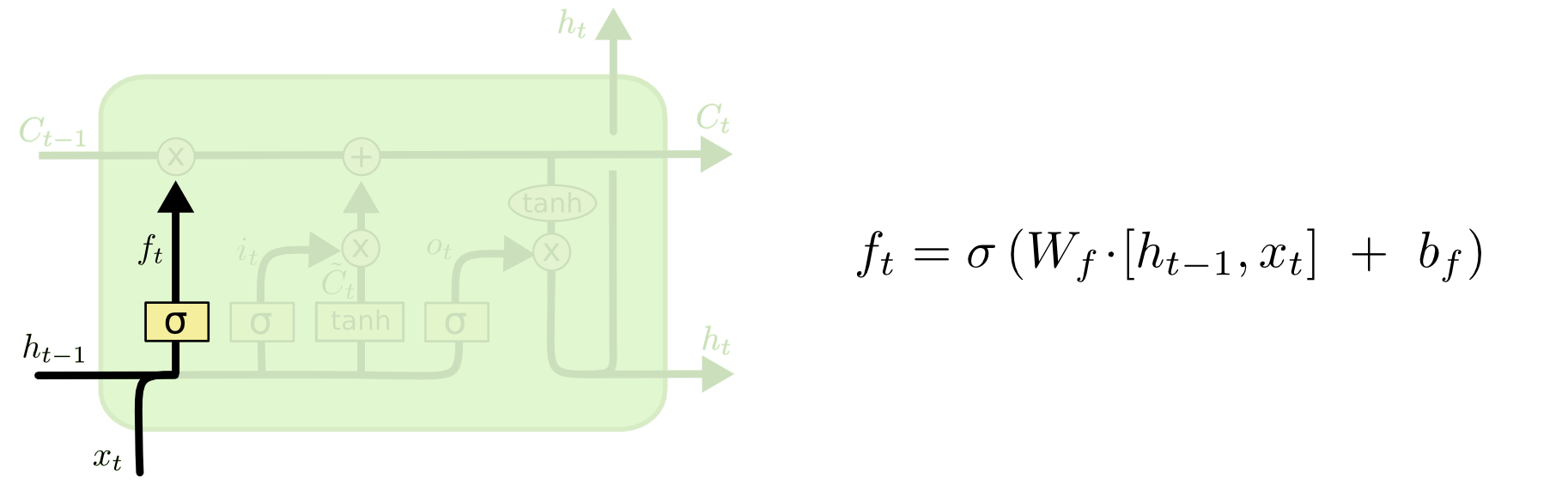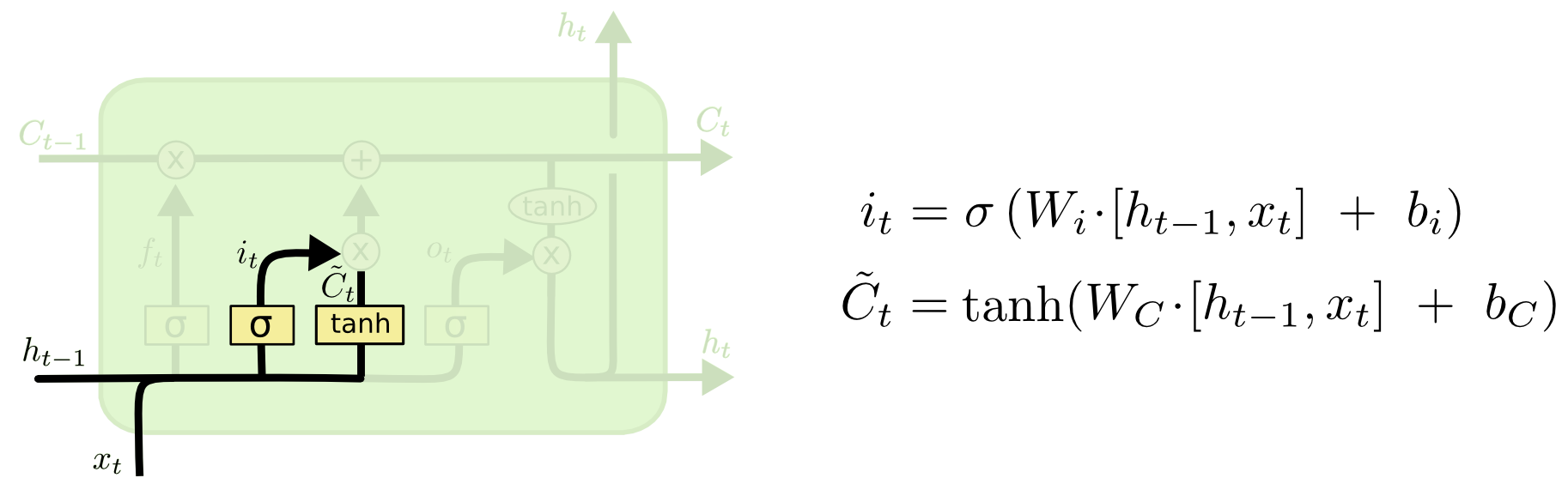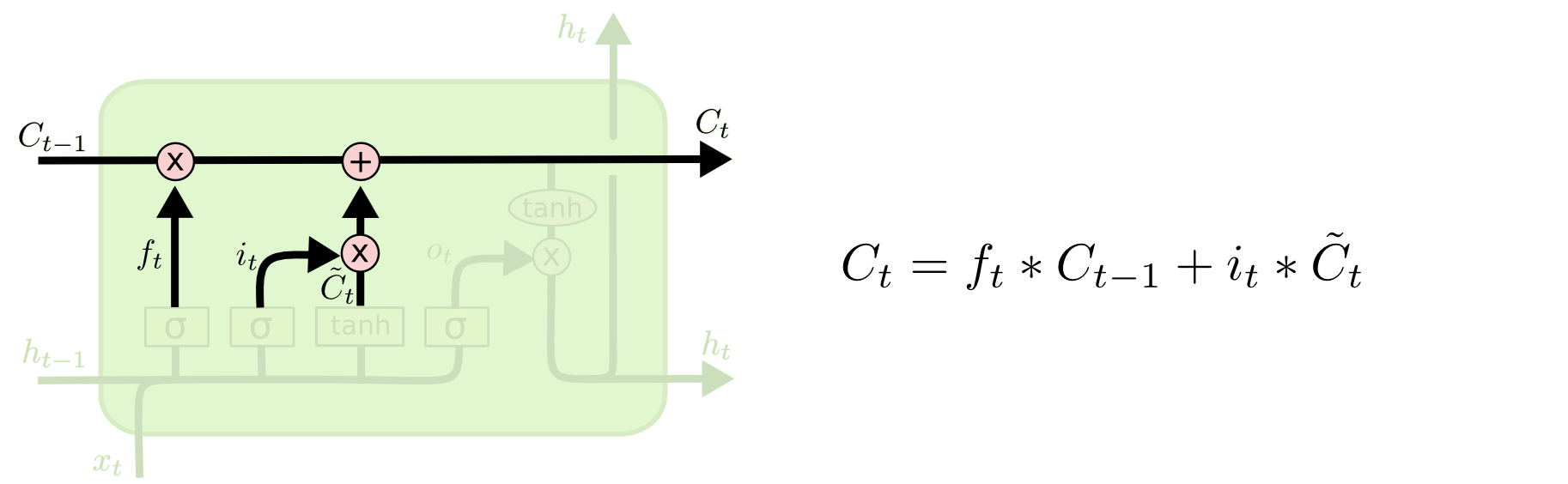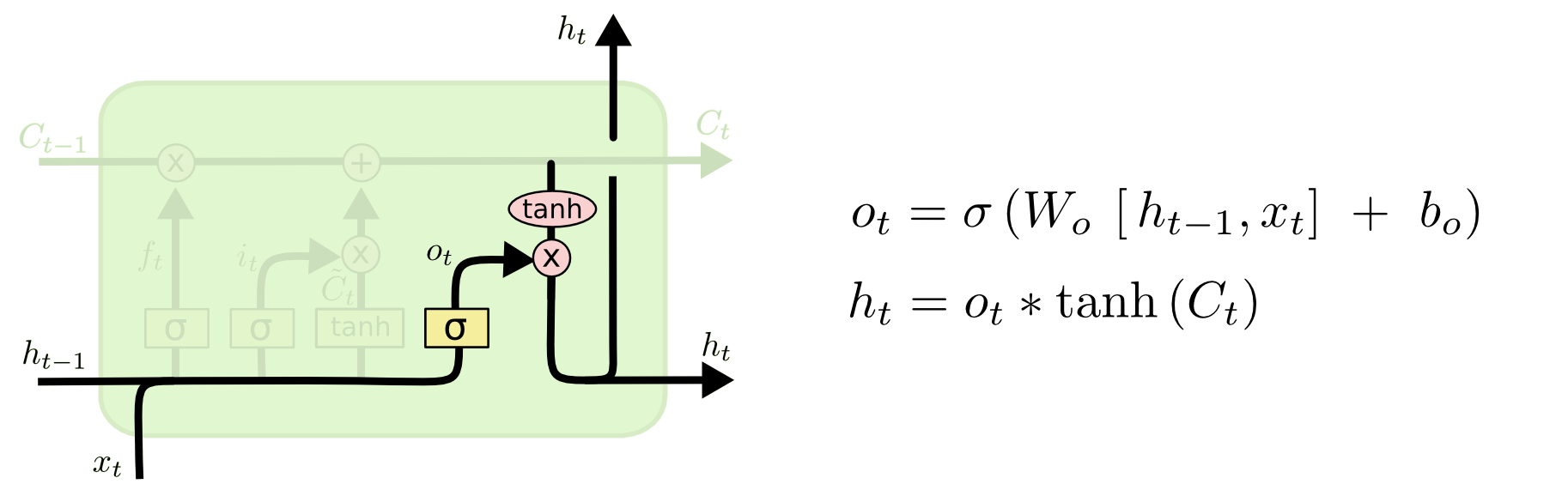### 2.3 LSTM 单元变体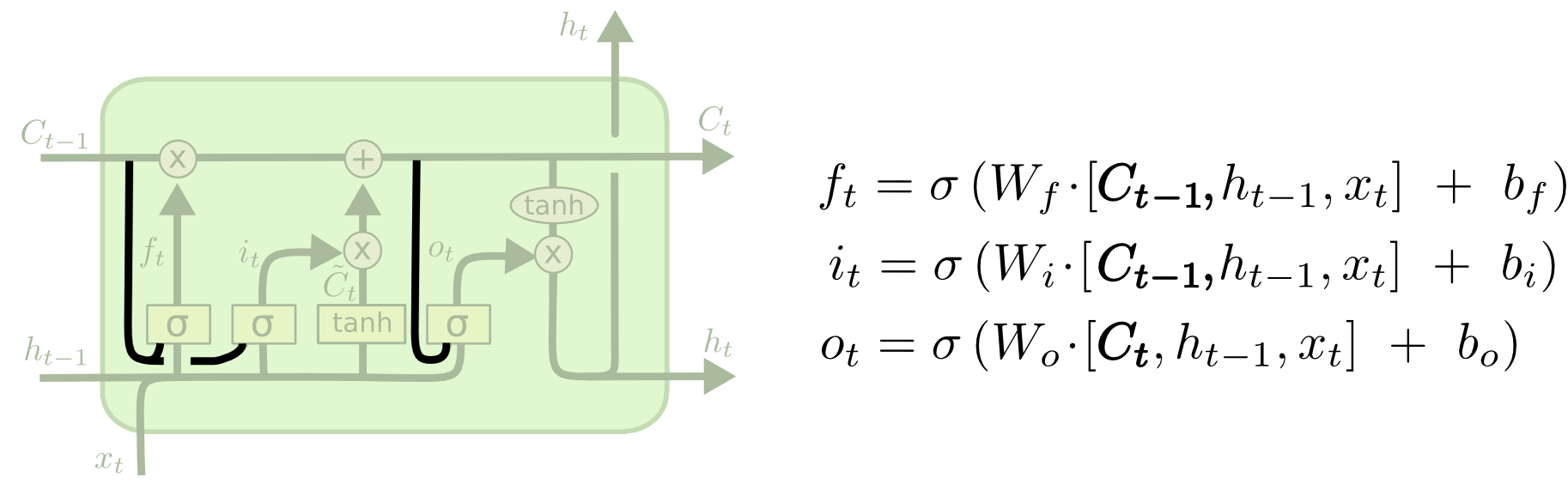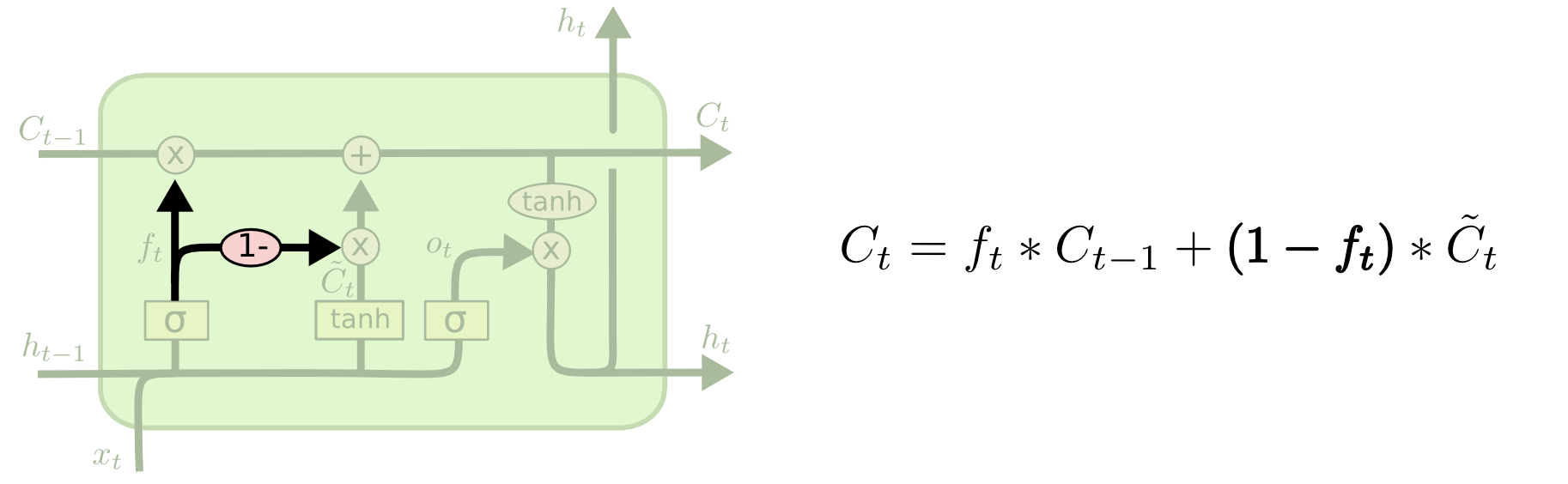## 3. 门控循环单元(Gated Recurrent Unit, GRU)

GRU4 是一种比 LSTM 稍简单一些的循环单元. GRU 把 LSTM 中的隐藏状态 $$h$$ 和单元状态 $$C$$ 合并为单个的隐藏状态. 如下图所示.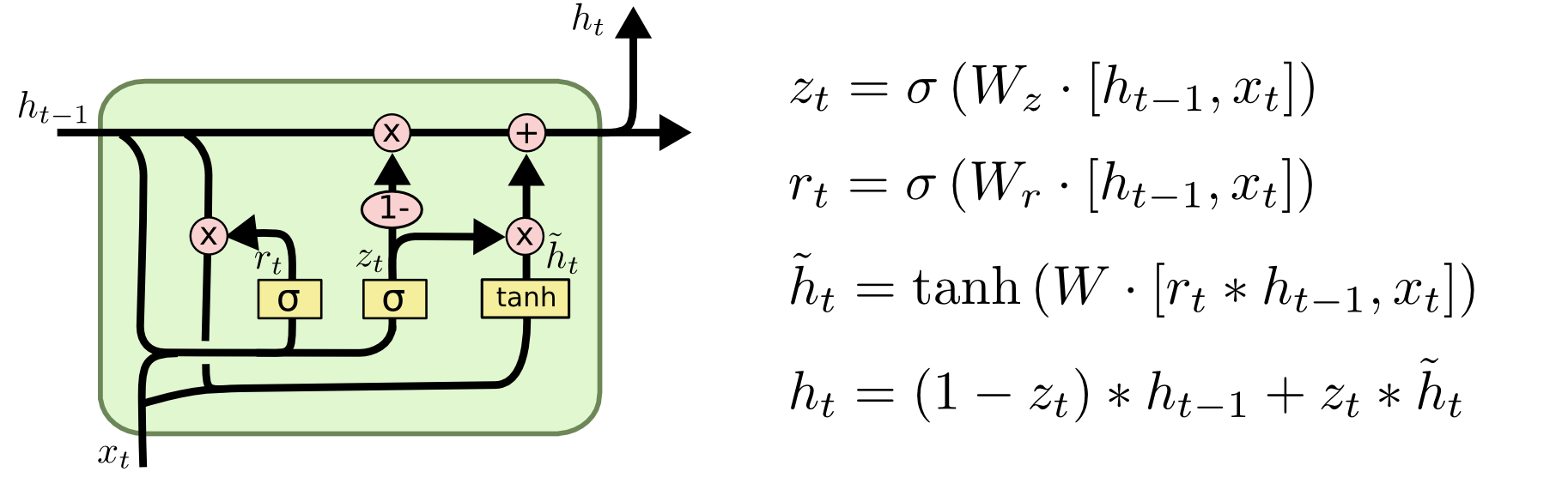## 4. 编码-解码器 (Encoder-Decoder)

$P(Y|X) = \prod_{t=1}^TP(y_t\lvert y_1, y_2, \cdots, y_{t-1}, X).$

$h_s = f_{enc}(h_{s-1}, x_s).$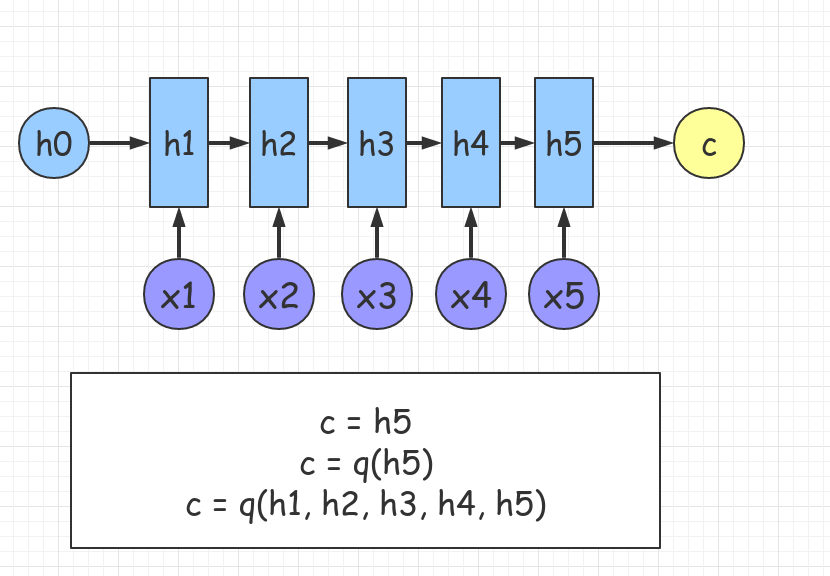\begin{align} g_1 &= h_s \\ g_t &= f_{dec}(g_{t-1}, y_{t-1}) \end{align}

$P(y_t\lvert y_1, y_2, \cdots, y_{t-1}, X) = Softmax(Linear(g_t)).$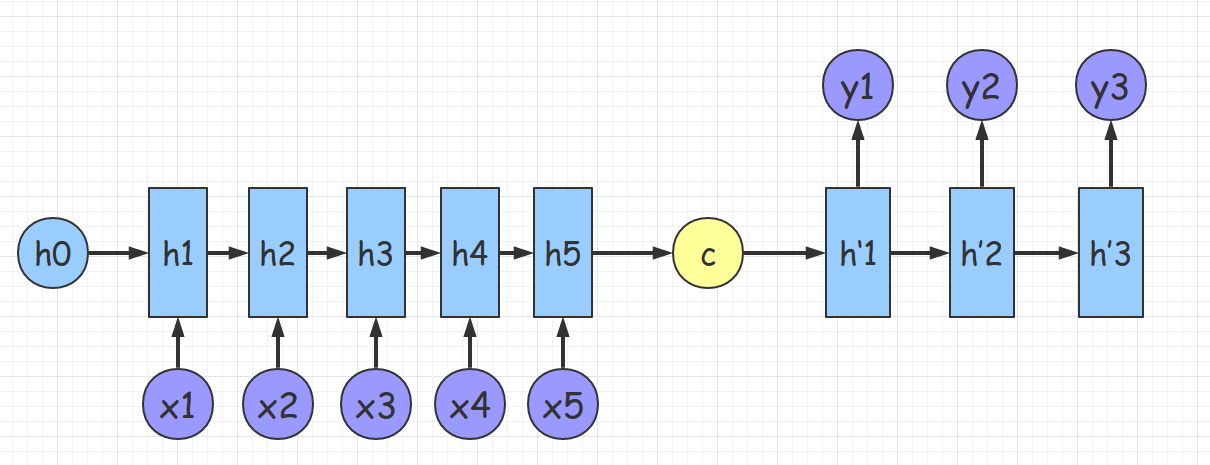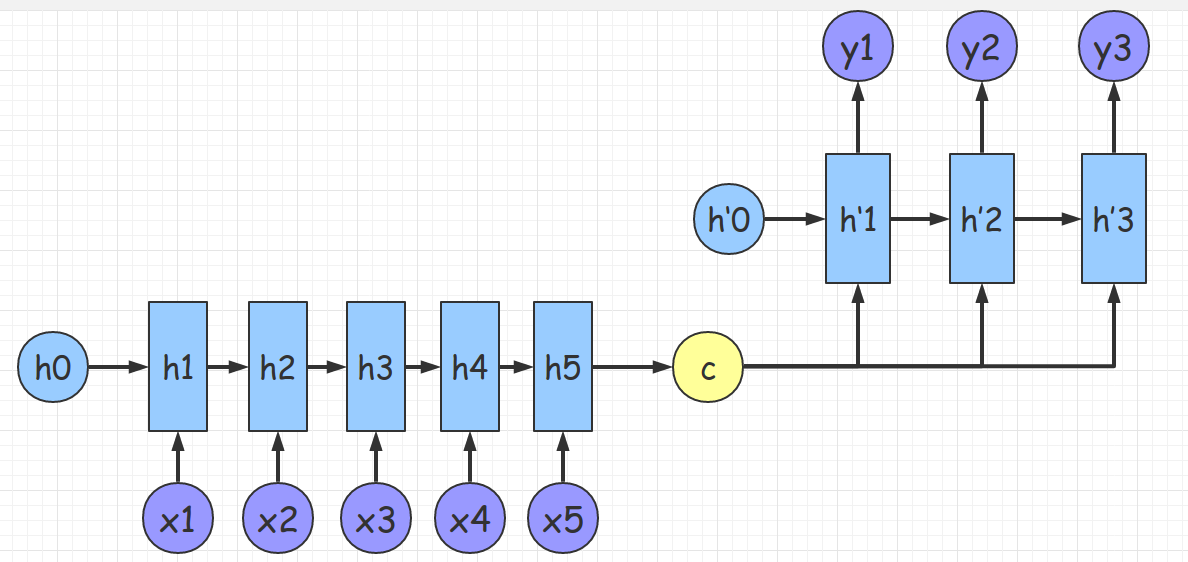### 4.1 编码-解码器模型的局限性

• 信息的丢失: 整个时间序列只能压缩为一个固定长度的语义向量
• 不合理性: seq2seq 的任务中输入序列 $$\{x_0, x_1, \dots, x_{t−1}, x_𝑡, x_{t+1},\dots \}$$ 中的每个元素对所有 $$y_s$$ 的贡献度是相同的

## 5. 注意力机制 (Attention Mechanism)9

### 5.1 什么是注意力?

Attention is the behavioral and cognitive process of selectively concentrating on a discrete aspect of information, whether deemed subjective or objective, while ignoring other perceivable information.

• 决定输入信息的哪部分是重要的
• 把资源集中分配到重要的信息上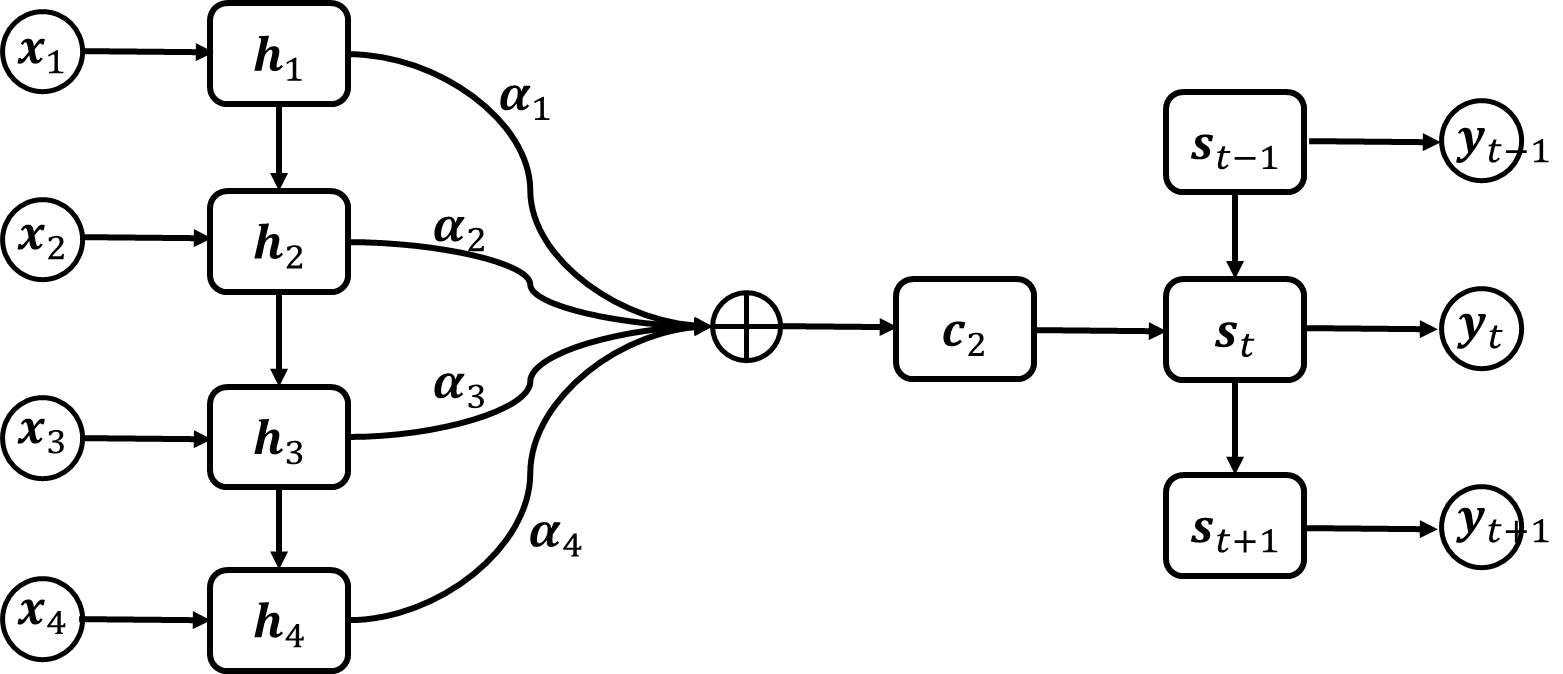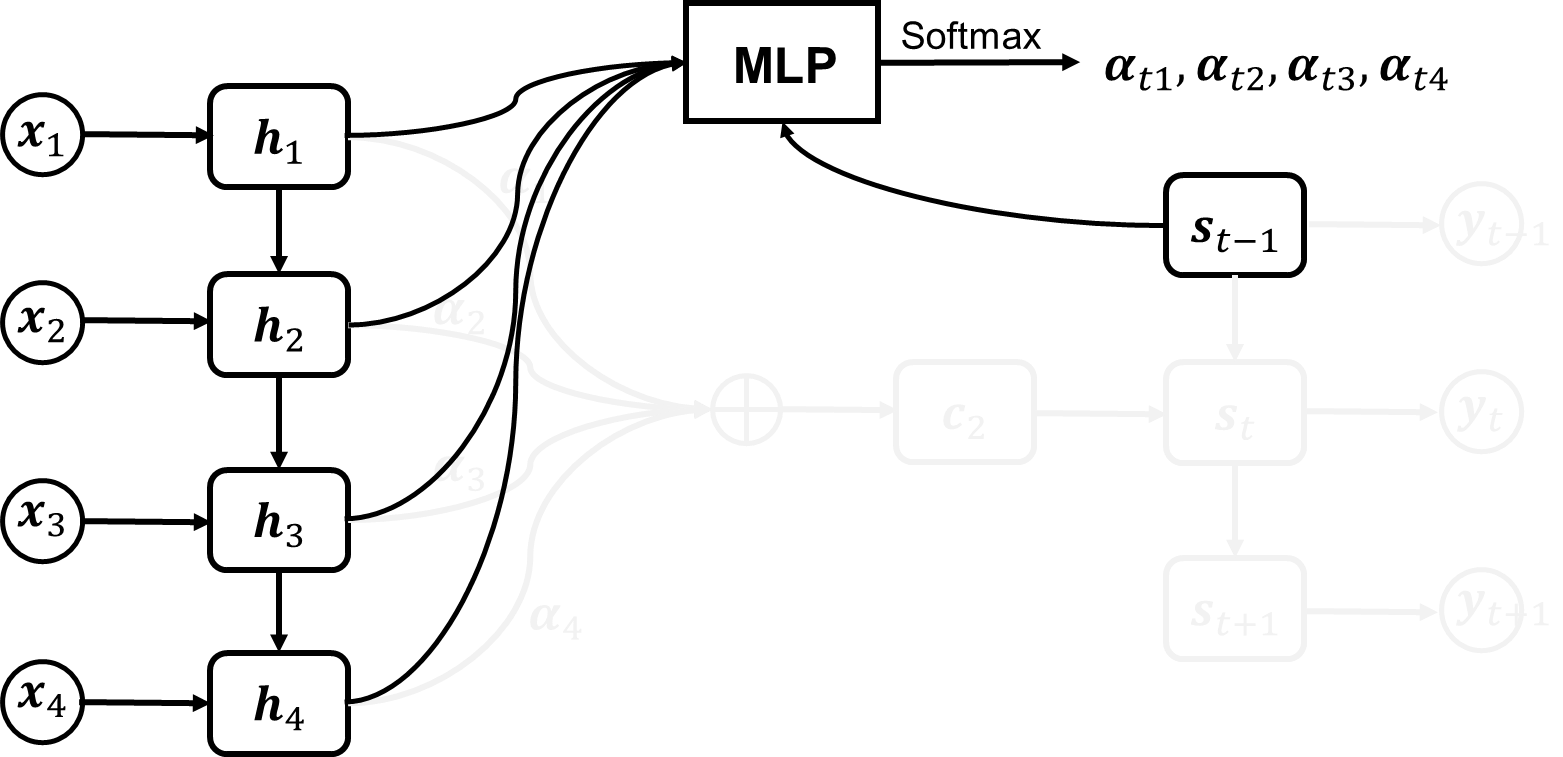### 5.2 自注意力 (Self-Attention)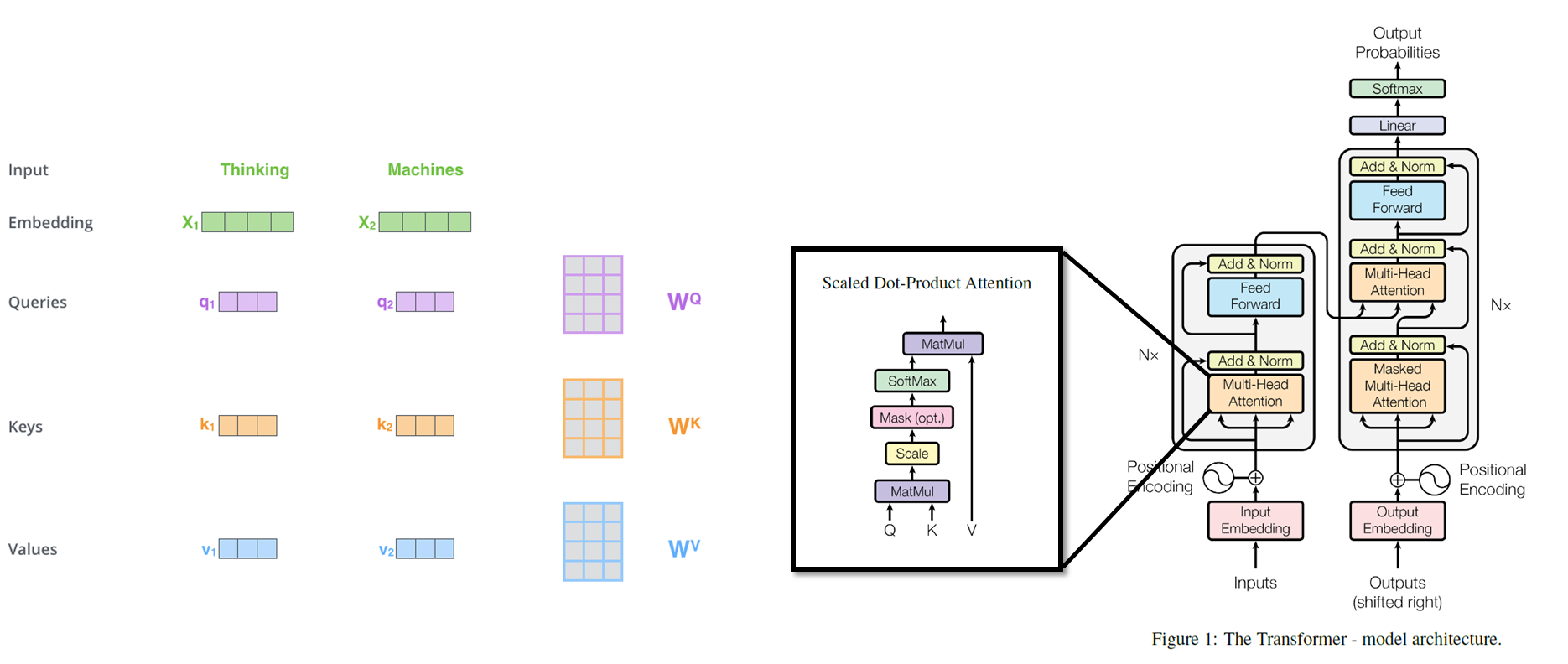• 输入的序列首先转化为相同长度的 embedding
• 创建三个矩阵: 查询矩阵 (Query), 键矩阵 (Key), 值矩阵 (Value)
• 使用这三个矩阵把每个单词的 embedding 映射为三个稍短的特征向量, 分别代表了当前单词的查询向量, 键向量和值向量.
• 比如我们要计算单词 Thinking 关于句子中其他单词的注意力时, 使用 Thinking 的查询向量依次与句子中所有单词 (包括 Thinking) 的键向量做点积来计算相似度, 并对相似度进行归一化得到加权系数 (这是 attention 的核心部分).
• 加权系数乘到所有单词的值向量上来得到单词 Thinking 经过自注意力模块后输出的特征向量, 这个特征向量中可以看作包含了翻译任务重对准确翻译 Thinking 所需要的信息, 而不包含其他信息 (其他信息在翻译其他词时可能有用, 但在翻译 Thinking 时无用).

## 6. tensorflow 官方代码解析

### 参数设置

1
2
3
4
5
6
7
8
9
10
11
12
13
14
15
16
17
flags = tf.flags
logging = tf.logging

flags.DEFINE_string(    # 定义变量 model的值为small, 后面的是注释
"model", "small",
"A type of model. Possible options are: small, medium, large.")

flags.DEFINE_string("data_path",   #定义下载好的数据的存放位置
"data_path")
flags.DEFINE_bool("use_fp16", False,    # 是否使用 float16格式？
"Train using 16-bit floats instead of 32bit floats")

FLAGS = flags.FLAGS     # 可以使用FLAGS.model来调用变量 model的值。

def data_type():
return tf.float16 if FLAGS.use_fp16 else tf.float321234567891011121314151617


1
2
3
4
5
6
7
8
9
10
11
12
init_scale = 0.1        # 相关参数的初始值为随机均匀分布，范围是[-init_scale,+init_scale]
learning_rate = 1.0     # 学习速率,在文本循环次数超过max_epoch以后会逐渐降低
num_layers = 2          # lstm层数
num_steps = 20          # 单个数据中，序列的长度。
hidden_size = 200       # 隐藏层中单元数目
max_epoch = 4           # epoch<max_epoch时，lr_decay值=1,epoch>max_epoch时,lr_decay逐渐减小
max_max_epoch = 13      # 指的是整个文本循环次数。
keep_prob = 1.0         # 用于dropout.每批数据输入时神经网络中的每个单元会以1-keep_prob的概率不工作，可以防止过拟合
lr_decay = 0.5          # 学习速率衰减
batch_size = 20         # 每批数据的规模，每批有20个。
vocab_size = 10000      # 词典规模，总共10K个词123456789101112


### PTBModel

• 多层LSTM结构的构建
• 输入预处理
• LSTM的循环
• 损失函数计算
• 梯度计算
• 修剪

#### 1.1 LSTM结构

1
2
3
4
5
6
7
8
9
class PTBInput(object):
"""The input data."""

def __init__(self, config, data, name=None):
self.batch_size = batch_size = config.batch_size
self.num_steps = num_steps = config.num_steps
self.epoch_size = ((len(data) // batch_size) - 1) // num_steps
data, batch_size, num_steps, name=name)		# 该类主要作用是这句, 从文件中读取数据


self.input_data 和 self.targets 都是 index 的序列, 尺寸为 [batch_size, num_steps]. 注意此时不论是input还是target都是用词典id来表示单词的。

PTBModel.__init__() 函数:

1
2
3
4
5
6
self._input = input_	# [batch_size, num_steps]

batch_size = input_.batch_size
num_steps = input_.num_steps
size = config.hidden_size		# 隐藏层规模
vocab_size = config.vocab_size	# 词典规模


1
2
3
4
5
6
7
8
9
10
def lstm_cell():
if 'reuse' in inspect.getargspec(tf.contrib.rnn.BasicLSTMCell.__init__).args:
return tf.contrib.rnn.BasicLSTMCell(size,
forget_bias=0.0,
state_is_tuple=True,
reuse=tf.get_variable_scope().reuse)
else:
return tf.contrib.rnn.BasicLSTMCell(size,
forget_bias=0.0,
state_is_tuple=True)#### 1.2 DropoutWrapper

1
2
3
4
attn_cell = lstm_cell
if is_training and config.keep_prob < 1:	# 在外面包裹 dropout
def attn_cell():
return tf.contrib.rnn.DropoutWrapper(lstm_cell(), output_keep_prob=config.keep_prob)#### 1.3 多层LSTM结构和状态初始化

1
2
3
4
cell = tf.contrib.rnn.MultiRNNCell([attn_cell() for _ in range(config.num_layers)],
state_is_tuple=True)
# 参数初始化,rnn_cell.RNNCell.zero_stat
self._initial_state = cell.zero_state(batch_size, data_type())


#### 2. 输入预处理

1
2
3
4
5
6
7
8
9
10
11
12
13
with tf.device("/cpu:0"):
# 把描述单词的指标 idx ([1, 1]) 变为 embedding ([1, hidden_size]) 描述
# 使用 embedding 描述可以让网络从描述中学习单词之间的关联, 否则单个的指标之间是独立的, 无法学习关联 ???
embedding = tf.get_variable("embedding", [vocab_size, size], dtype=data_type())

# 将输入的每个 sequence ([batch_size, num_steps]) 用 embedding 表示
# shape = [batch_size, num_steps, hidden_size]
# 所以每个 x_t 都是一个 batch_size x 1 x hidden_size 的向量
# 在程序里 1 自动省去, 所以每个 x_t 实际上是 [batch_size, hidden_size] 的向量
inputs = tf.nn.embedding_lookup(embedding, input_.input_data)

if is_training and config.keep_prob < 1:
inputs = tf.nn.dropout(inputs, config.keep_prob)


1
2
3
4
5
6
7
8
9
10
11
12
13
14
15
16
17
18
19
20
21
22
23
24
25
# 示例代码
import tensorflow as tf
import numpy as np

sess = tf.InteractiveSession()

embedding = tf.Variable(np.identity(5,dtype=np.int32))
input_ids = tf.placeholder(dtype=tf.int32,shape=[None])
input_embedding = tf.nn.embedding_lookup(embedding,input_ids)

sess.run(tf.initialize_all_variables())
print(sess.run(embedding))
#[[1 0 0 0 0]
# [0 1 0 0 0]
# [0 0 1 0 0]
# [0 0 0 1 0]
# [0 0 0 0 1]]
print(sess.run(input_embedding,feed_dict={input_ids:[1,2,3,0,3,2,1]}))
#[[0 1 0 0 0]
# [0 0 1 0 0]
# [0 0 0 1 0]
# [1 0 0 0 0]
# [0 0 0 1 0]
# [0 0 1 0 0]
# [0 1 0 0 0]]


#### 3. LSTM循环

1
2
3
4
5
6
7
8
9
10
11
12
13
outputs = []
state = self._initial_state # state 表示 各个batch中的状态
with tf.variable_scope("RNN"):
for time_step in range(num_steps):
if time_step > 0: tf.get_variable_scope().reuse_variables()
# 输入: [batch_size, hidden_size]
# 按照顺序向cell输入文本数据, cell_out: [batch_size, hidden_size]
(cell_output, state) = cell(inputs[:, time_step, :], state)
outputs.append(cell_output)  # output: [num_steps][batch_size, hidden_size]

# 把之前的list展开，成 [batch, num_steps, hidden_size]
# 然后 reshape 成 [batch*numsteps, hidden_size], 这是为了后面 softmax 层计算方便
output = tf.reshape(tf.stack(axis=1, values=outputs), [-1, size])


#### 4. 损失函数计算

1
2
3
4
5
6
7
8
9
10
11
12
13
14
15
16
# softmax_w , shape=[hidden_size, vocab_size], 用于将distributed表示的单词转化为one-hot表示
softmax_w = tf.get_variable("softmax_w", [size, vocab_size], dtype=data_type())
softmax_b = tf.get_variable("softmax_b", [vocab_size], dtype=data_type())
# [batch*numsteps, vocab_size] 从隐藏语义转化成完全表示
logits = tf.matmul(output, softmax_w) + softmax_b

# loss , shape=[batch*num_steps], 带权重的交叉熵计算
loss = tf.contrib.seq2seq.sequence_loss(
logits,					# [batch*numsteps, vocab_size]
input_.targets,			# [batch_size, num_steps]
tf.ones([batch_size, num_steps], dtype=data_type()),	# weight
average_across_timesteps=False,
average_across_batch=True			# loss = loss / batch_size
)
self._cost = cost = tf.reduce_sum(loss)
self._final_state = state


#### 5. 梯度计算

1
2
self._lr = tf.Variable(0.0, trainable=False)  # lr 指的是 learning_rate
tvars = tf.trainable_variables()


1
2


1
2
3
4
5
6
7
xs,
aggregation_method=None):


$g_i = \sum_{j=0}^{len(y)}\frac{\partial y_i}{\partial x_i} \\ g = [g_0, g_1, \cdots, g_{len(x)}$

#### 6. 梯度修剪

tf.clip_by_global_norm 修正梯度值，用于控制梯度爆炸的问题。梯度爆炸和梯度弥散的原因一样，都是因为链式法则求导的关系，导致梯度的指数级衰减。为了避免梯度爆炸，需要对梯度进行修剪。 先来看这个函数的定义：

1
def clip_by_global_norm(t_list, clip_norm, use_norm=None, name=None):


$$L_c^i = \frac{L_t^i N_c}{\max(N_c, N_g)},\quad N_g = \sqrt{\sum_i(L_t^i)^2}$$ 其中, $$L_t^i$$ 和 $$L_c^i$$ 代表 t_list[i]list_clipped[i], $$N_c$$ 和 $$N_g$$ 代表 clip_norm 和 global_norm 的值。

#### 7. 优化参数

1
2
3
4
5
6
7
# 梯度下降优化，指定学习速率
global_step=tf.contrib.framework.get_or_create_global_step())  # 将梯度应用于变量


### 8. Main 函数

1
2
3
4
5
6
7
8
initializer = tf.random_uniform_initializer(-config.init_scale, config.init_scale)

with tf.name_scope("Train"):
train_input = PTBInput(config=config, data=train_data, name="TrainInput")
with tf.variable_scope("Model", reuse=None, initializer=initializer):
m = PTBModel(is_training=True, config=config, input_=train_input)
tf.summary.scalar("Training Loss", m.cost)
tf.summary.scalar("Learning Rate", m.lr)


1. Understanding LSTM Networks – Colah’s blogs

2. Long short-term memory
Hochreiter S, Schmidhuber J.
[link] In Neural computation, 1997, 9(8): 1735-1780.

3. Recurrent nets that time and count
Gers F A, Schmidhuber J.
[link] In Proceedings of the IEEE-INNS-ENNS International Joint Conference on Neural Networks. 2000.

4. Learning phrase representations using RNN encoder-decoder for statistical machine translation
Cho K, Van Merriënboer B, Gulcehre C, et al.
[link] In arXiv preprint arXiv:1406.1078, 2014.  2

5. Order Matters: Sequence to Sequence for Sets
Vinyals, Oriol, Samy Bengio, and Manjunath Kudlur.

6. 详解从 Seq2Seq模型、RNN结构、Encoder-Decoder模型 到 Attention模型

7. Learning phrase representations using RNN encoder-decoder for statistical machine translation
Cho K, Van Merriënboer B, Gulcehre C, et al.
[link] In arXiv preprint arXiv:1406.1078, 2014.

8. Sequence to Sequence Learning with Neural Networks
Ilya Sutskever, Oriol Vinyals, Quoc V.Le.

9. Neural machine translation by jointly learning to align and translate
Dzmitry Bahdanau, Kyunghyun Cho, Yoshua Bengio